Win up to 100% scholarship on Aakash BYJU'S JEE/NEET courses with ABNAT Win up to 100% scholarship on Aakash BYJU'S JEE/NEET courses with ABNAT

# Mutual Inductance

Mutual inductance is the main operating principle of generators, motors and transformers. Any electrical device having components that tend to interact with another magnetic field also follows the same principle. The interaction is usually brought about by a mutual induction where the current flowing in one coil generates a voltage in a secondary coil.

## What Is Mutual Inductance?

When two coils are brought in proximity to each other, the magnetic field in one of the coils tends to link with the other. This further leads to the generation of voltage in the second coil. This property of a coil which affects or changes the current and voltage in a secondary coil is called mutual inductance.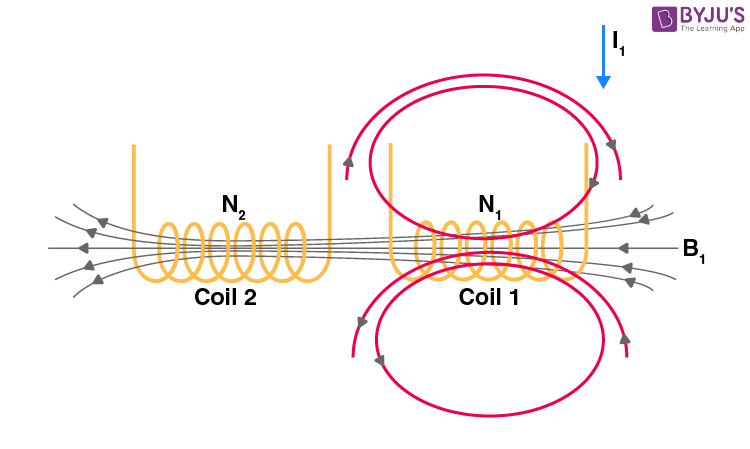Changing I1 produces changing magnetic flux in coil 2.

In the first coil of N1 turns, when a current I1 passes through it, magnetic field B is produced. As the two coils are closer to each other, a few magnetic field lines will also pass through coil 2.

$$\begin{array}{l}{{\phi }_{21}}\to\ \text{magnetic flux in one turn of coil 2 due to current}\ I_1.\end{array}$$

If we vary the current with respect to time, then there will be an induced emf in coil 2.

$$\begin{array}{l}{{\varepsilon }_{ind}}=-\frac{d\phi }{dt}\end{array}$$

$$\begin{array}{l}{{\varepsilon }_{21}}=-{{N}_{2}}\frac{d{{\phi }_{21}}}{dt}\end{array}$$

$$\begin{array}{l}{{\varepsilon }_{21}}=-{{N}_{2}}\frac{d}{dt}\left( \overline{B}.\,\overline{A} \right)\end{array}$$

The induced emf in coil 2 is directly proportional to the current that passes through coil 1.

$$\begin{array}{l}{{N}_{2}}\,{{\phi }_{21}}\propto {{I}_{1}}\end{array}$$

$$\begin{array}{l}{{N}_{2}}\,{{\phi }_{21}}={{M}_{21}}{{I}_{1}}….(1)\end{array}$$

The constant of proportionality is called mutual inductance. It can be written as

$$\begin{array}{l}{{M}_{21}}=\frac{{{N}_{2}}{{\phi }_{21}}}{{{I}_{1}}}….(2)\end{array}$$

The SI unit of inductance is henry (H)

$$\begin{array}{l}1H=\frac{1(\text{Tesla}).1\left( {{m}^{2}} \right)}{1\,\,A}\end{array}$$

In a similar manner, the current in coil 2, I2, can produce an induced emf in coil 1 when I2 varies with respect to time. Then,

$$\begin{array}{l}{{\varepsilon }_{12}}=-{{N}_{1}}\frac{d{{\phi }_{12}}}{dt}\end{array}$$

$$\begin{array}{l}{{N}_{1}}{{\phi }_{12}}\propto {{I}_{2}}\end{array}$$

$$\begin{array}{l}{{N}_{1}}{{\phi }_{12}}={{M}_{12}}{{I}_{2}}….(3)\end{array}$$

$$\begin{array}{l}{{M}_{12}}=\frac{{{N}_{1}}{{\phi }_{12}}}{{{I}_{2}}}….(4)\end{array}$$

This constant of proportionality is another mutual inductance.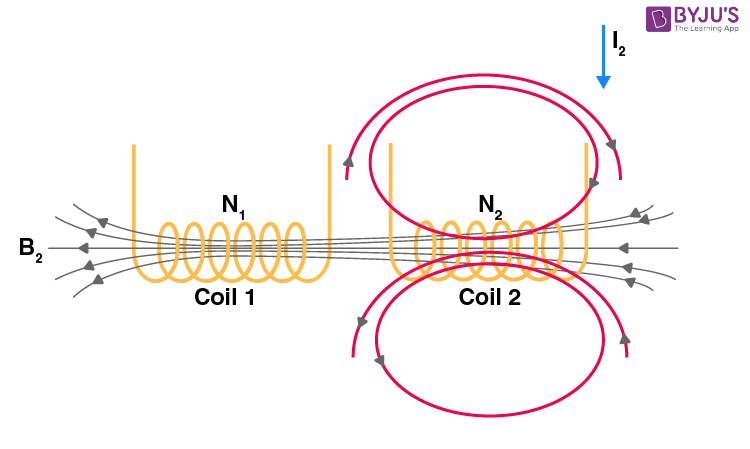Changing I2 produces changing magnetic flux in coil 1.

## Reciprocity Theorem

Experiments and calculations that combine Ampere’s law and Biot-Savart’s law confirm that the two constants, M21 and M12, are equal in the absence of material medium between the two coils.

M12 = M21 … (5)

This property is called reciprocity, and by using the reciprocity theorem, we can simply write the mutual inductance between two coils as,

$$\begin{array}{l}{{\text{M}}_{12}}\text{=}\,{{\text{M}}_{21}}\equiv \text{M}\end{array}$$

## EMF of Mutual Inductance

Considering the mutual inductance between the two coils we just discussed, we defined mutual inductance M21 of coil 2 with respect to 1 as,

$$\begin{array}{l}{{\text{M}}_{21}}\text{=}\,\frac{{{N}_{2}}{{\phi }_{21}}}{{{I}_{1}}}\end{array}$$

$$\begin{array}{l}{{\text{M}}_{21}}{{I}_{1}}={{N}_{2}}{{\phi }_{21}}\end{array}$$

If I1 changes with time,

$$\begin{array}{l}{{M}_{21}}\frac{d{{I}_{1}}}{dt}={{N}_{2}}\frac{d{{\phi }_{21}}}{dt}….(6)\end{array}$$

According to Faraday’s law of induction,

$$\begin{array}{l}{{\varepsilon }_{ind}}=-\frac{d\phi }{dt}\end{array}$$

Thus, induced emf in coil 2 due to the current in coil 1 is given by

$$\begin{array}{l}{{\varepsilon }_{2}}=-{{M}_{21}}\frac{d{{I}_{1}}}{dt}….(7)\end{array}$$

Similarly, induced emf in coil 1 due to changing current in coil 2 can be given as,

$$\begin{array}{l}{{\varepsilon }_{1}}=-{{M}_{12}}\frac{d{{I}_{2}}}{dt}….(8)\end{array}$$

From experiments (equation (5),

M21 = M12 = M

Therefore,

$$\begin{array}{l}{{\varepsilon }_{1}}=-M\frac{d{{I}_{2}}}{dt}\,\,\,\,\,\,{{\varepsilon }_{2}}=-M\frac{d{{I}_{1}}}{dt}\end{array}$$

The coefficient of mutual induction – mutual inductance depends only on the geometrical factor of the two coils, such as the number of turns and radii of two coils and on the properties of a material medium, such as the magnetic permeability of the medium surrounding the coils.

## How to Find Mutual Inductance?

Steps to find mutual inductance (M).

(i) Assume current in one of the coils (say I1 in coil 1)

(ii) Deduce the expression for the magnetic field in the neighbouring coil (2) due to I1.

(iii) Write the flux linkage equation.

$$\begin{array}{l}{{N}_{2}}{{\phi }_{2}}=M{{I}_{1}}…..(9)\end{array}$$

(iv) Obtain the magnetic flux linked,

$$\begin{array}{l}{{N}_{2}}\left( {{\phi }_{2}} \right)={{N}_{2}}\left( {{B}_{1}}.\,{{A}_{2}} \right)…..(10)\end{array}$$

(v) Compare the above two equations and find mutual inductance, M.

### Mutual Inductance Problems

(1) Mutual induction between circular coils.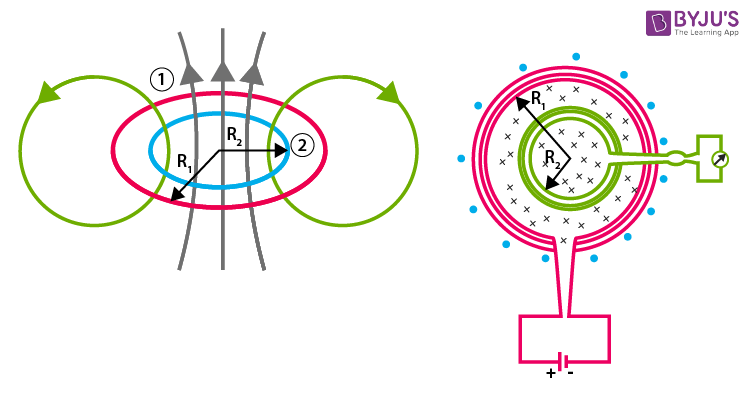Consider two circular coils (closely packed) coaxially placed to each other. The coil with a larger radius has N1 turns, and that with a smaller radius has N2 turns. Also, assume that R1>> R2.

The above-mentioned calculation is the same for the following case as well.

• Mutual inductance of two concentric coplanar loops.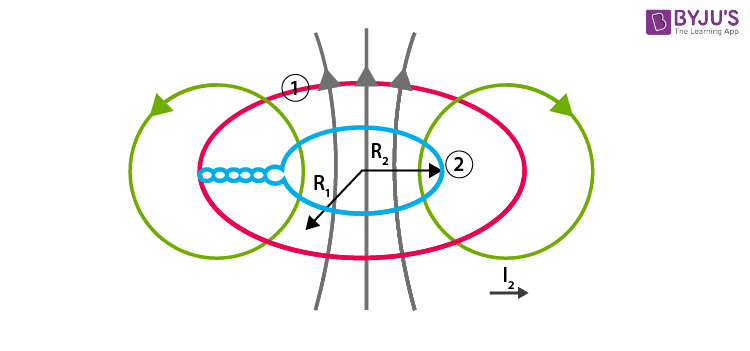• Mutual induction between two solenoids.

## Mutual Inductance of a Coaxial Solenoid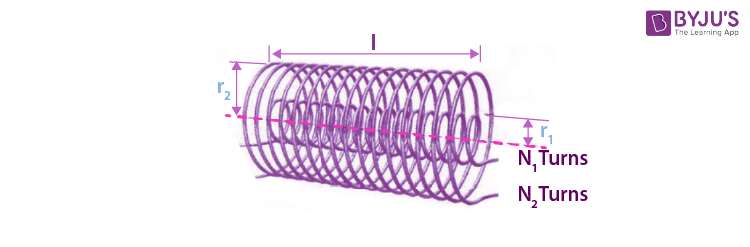Consider two coaxial solenoids, of which the outer solenoid S2 has radius r2, and N2 turns, whereas the inner solenoid S1 has radius r1 and N1 turns. Both the solenoids are of equal length.

When there is a current I2 in the solenoid S2, the magnetic induction due to I2 is given by,

$$\begin{array}{l}{{B}_{2}}={{\mu }_{0}}{{n}_{2}}{{I}_{2}}={{\mu }_{0}}\frac{{{N}_{2}}}{l}{{I}_{2}}.\end{array}$$

The corresponding flux linkage with solenoid S1 is,

$$\begin{array}{l}{{N}_{1}}{{\phi }_{12}}={{N}_{1}}{{B}_{2}}.\,{{A}_{1}}\end{array}$$

$$\begin{array}{l}{{N}_{1}}{{\phi }_{12}}={{N}_{1}}{{\mu }_{0}}\frac{{{N}_{2}}}{l}{{I}_{2}}.\,\pi r_{1}^{2}….(11)\end{array}$$

Also,

$$\begin{array}{l}{{N}_{1}}{{\phi }_{12}}={{M}_{12}}{{I}_{2}}….(12)\end{array}$$

On comparing (11) and (12),

$$\begin{array}{l}{{M}_{12}}=\frac{{{\mu }_{0}}{{N}_{1}}{{N}_{2}}\pi \,\,r_{1}^{2}}{l}\end{array}$$

Similarly, when a current I1 is set up through S1, then the magnetic flux linked in S2 is given by,

$$\begin{array}{l}{{N}_{2}}{{\phi }_{21}}={{M}_{21}}{{I}_{1}}….(13)\end{array}$$

Note that the magnetic flux due to current I1 in S1 is assumed to be confined only inside the solenoid S1. Also, the solenoids are very long compared to their radii, and the flux linkage in S2 is

$$\begin{array}{l}{{N}_{2}}{{\phi }_{21}}={{N}_{2}}\left( {{B}_{1}}\,.\,\,{{A}_{1}} \right)\end{array}$$
→ (Area confined within S1.)

$$\begin{array}{l}{{N}_{2}}{{\phi }_{21}}={{N}_{2}}{{\mu }_{0}}\frac{{{N}_{1}}}{l}.\,{{I}_{1}}\,.\,\,\pi \,r_{1}^{2}….(14)\end{array}$$

From (13) and (14),

$$\begin{array}{l}{{M}_{21}}={{\mu }_{0}}\frac{{{N}_{1}}{{N}_{2}}}{l}\,\pi \,\,r_{1}^{2}={{M}_{12}}=M\end{array}$$

Example Problem: Calculate the mutual inductance between a solenoid of length l and cross-sectional area A with N1 turns, and a circular coil of N2 turns that is wound at the centre of the solenoid.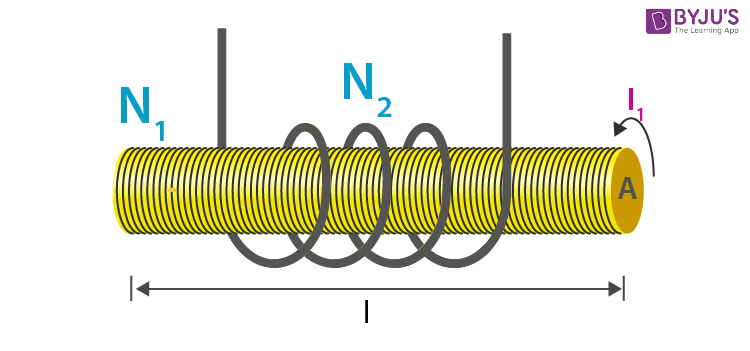Solution:

(i) Assume current I1 in the solenoid. The magnetic field B at its centre is given by

$$\begin{array}{l}B={{\mu }_{0}}n\,i={{\mu }_{0}}\frac{{{N}_{1}}}{l}{{I}_{1}}\end{array}$$

(ii) Magnetic flux linked with the small coil of area A is due to magnetic field B, then,

$$\begin{array}{l}{{N}_{2}}{{\phi }_{21}}={{N}_{2}}\left( B.\,A \right)\end{array}$$

$$\begin{array}{l}{{N}_{2}}{{\phi }_{21}}={{N}_{2}}.\,{{\mu}_{0}}\frac{{{N}_{1}}}{l}{{I}_{1}}\,\,.\,\,A….(a)\end{array}$$

(iii)

$$\begin{array}{l}{{N}_{2}}{{\phi }_{21}}=M\,\,{{I}_{1}}….(b)\end{array}$$

Equating (a) and (b),

$$\begin{array}{l}M={{\mu }_{0}}\frac{{{N}_{1}}{{N}_{2}}}{l}A\end{array}$$

## Applications of Mutual Inductance

The principle of mutual inductance is followed in various electronic devices. Some of them are as follows:

### Motors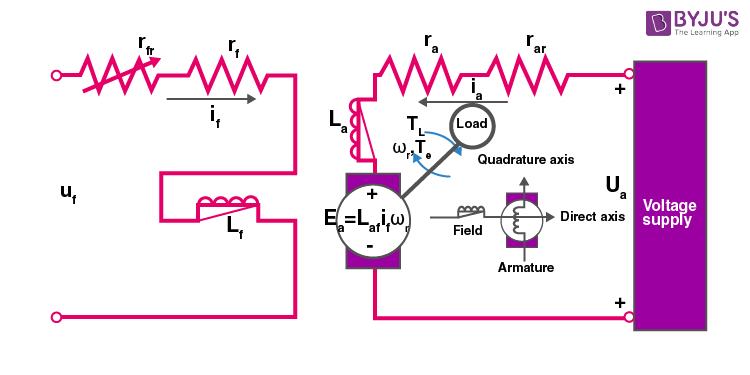Note the inductors (Lf and La) in the dc motor circuit that are mutually inducted.

### Transformer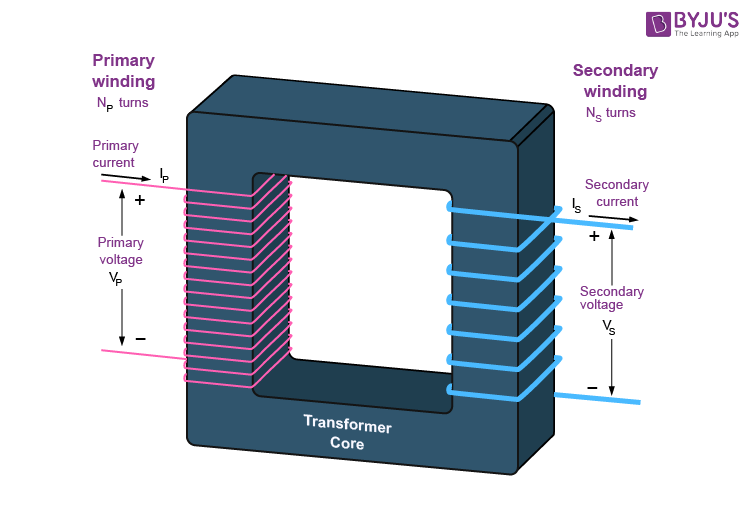### Generators

The induced EMG in a generator by electromagnetic induction is shown below. The direction of induced emf is given by Lenz law.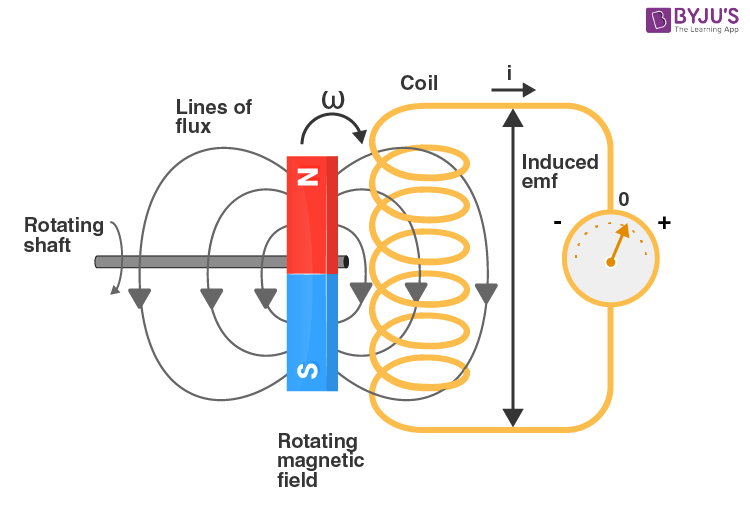When an electrical component (coil) is interacting or being influenced by the magnetic field in the neighbouring component, mutual inductance arises. The current flowing in one coil induces an emf in the neighbouring coil.

Solved Problems:

1. Two circular coils can be arranged in any of the three situations in the figure.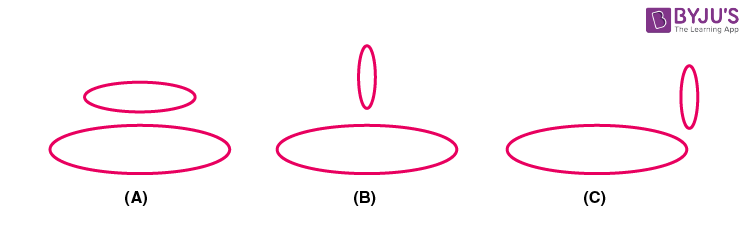The mutual inductance will be

(a) maximum in the situation (C)

(b) maximum in the situation (B)

(c) maximum in the situation (A)

(d) Same in all situations

Solution:

The mutual inductance will be maximum when maximum field lines due to one coil pass through the other. In such a situation, the magnetic flux linked will be maximum.

Therefore, in situation A, both coils are parallel to each other. Hence, more flux will be linked. Therefore, the mutual inductance will be larger.

2. Two coaxial coils are very close to each other, and their mutual inductance is 5 mH. If a current (50 A) sin 500 t is passed in one of the coils, then find the peak value of induced emf in the secondary coil.

(a) 50V (b) 500V (c) 125V (d) 250V

Solution:

Given:

$$\begin{array}{l}i=50\,\sin 500t\end{array}$$

emf of mutual induction is given by

$$\begin{array}{l}\varepsilon =-M\frac{di}{dt}\end{array}$$

$$\begin{array}{l}=-\left( 5\times {{10}^{-3}} \right)\frac{d}{dt}\left( 50\sin 500t \right)\end{array}$$

$$\begin{array}{l}=-5\times {{10}^{-3}}\times 50\cos 500t\times 500\end{array}$$

$$\begin{array}{l}\varepsilon =-125\cos 500t\end{array}$$

The peak value is 125V. Option c is correct.

## Frequently Asked Questions on Mutual Inductance

Q1

### What is the induced emf in a stationary circular coil kept in a uniform magnetic field?

As the coil is stationary in a uniform magnetic field, there is no change in magnetic flux generated. Hence, there is no induced emf in the coil.

Q2

### Explain how mutual inductance between a pair of circular coils changes when a sheet of iron is placed in between them. Assume all the other factors remain constant.

We know that the mutual inductance is directly proportional to the permeability of the medium surrounding the coils. When the permeability of the medium is increased by inserting a sheet of iron, then the mutual inductance between the coils also increases.

Q3

### When two spherical balls, one made up of copper and the other made up of glass, are allowed to fall freely under gravity from the same height above the ground, which one of the balls will reach the ground fast? Why?

As we know that acceleration due to gravity does not depend on the mass of the falling objects, the glass ball will reach the ground first. Because, being an insulator, glass is not affected by the earth’s magnetic field. Hence, there is no induced emf in the glass ball. Whereas in a copper ball, emf induced by the earth’s magnetic field will affect the fall of the ball. Thus, it falls slower.

Q4

### What are the factors that affect mutual inductance?

Mutual inductance between two coils is affected by the following:
Area of cross-section
Number of turns in each coil
Space between the two coils
Permeability of medium between the two coils
Length (in the case of the solenoid)

Q5

### How is the mutual inductance of a pair affected when the separation between the coils is increased?

When the separation between the coils is increased, the magnetic flux linked with the secondary coil decreases. Therefore, the mutual inductance of the pair of coils decreases.

Q6

### What happens when the number of turns in each coil is increased?

When there is an increase in the number of turns of each coil, mutual inductance increases.

Q7

### What is the application of mutual inductance?

Mutual inductance can be used in transformers, generators and electric motors.

Test Your Knowledge On Mutual Inductance!